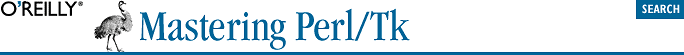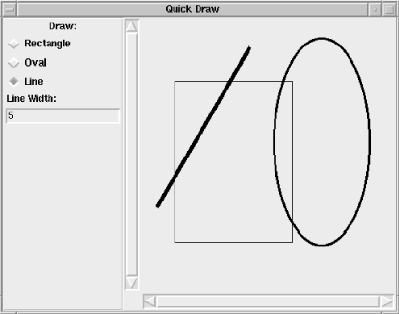home | O'Reilly's CD bookshelfs | FreeBSD | Linux | Cisco | Cisco Exam## 9.21. A Drawing Program Example

The Canvas widget is very versatile and can be useful for displaying different types of items. One of the first things that comes to mind when people think of a Canvas is a drawing program. To save you the trouble, here is a rudimentary drawing program called Quick Draw you can use to draw rectangles, ovals, and lines. You can also change the thickness of the objects before you draw them. It requires only a tiny bit of error-checking to make it a slicker program. Here's the code:

```use Tk;

\$mw = MainWindow->new;
\$mw->title("Quick Draw");

\$f = \$mw->Frame(-relief => 'groove',
-bd => 2,
-label => "Draw:")->pack(-side => 'left', -fill => 'y');
\$draw_item = "rectangle";
-text => "Rectangle",
-value => "rectangle",
-command => \&bind_start)->pack(-anchor => 'w');
-text => "Oval",
-value => "oval",
-command => \&bind_start)->pack(-anchor => 'w');
-text => "Line",
-value => "line",
-command => \&bind_start)->pack(-anchor => 'w');
\$f->Label(-text => "Line Width:")->pack(-anchor => 'w');
\$thickness = 1;
\$f->Entry(-textvariable => \\$thickness)->pack(-anchor => 'w');

\$c = \$mw->Scrolled("Canvas", -cursor => "crosshair")->pack(
-side => "left", -fill => 'both', -expand => 1);
\$canvas = \$c->Subwidget("canvas");

&bind_start( );

MainLoop;

sub bind_start {
# If there is a "Motion" binding, we need to allow the user
# to finish drawing the item before rebinding Button-1
# this fcn gets called when the finish drawing the item again
@bindings = \$canvas->bind("<Motion>");
return if (\$#bindings >= 0);

if (\$draw_item eq "rectangle"||\$draw_item eq "oval"||\$draw_item eq "line") {
\$canvas->bind("<Button-1>", [\&start_drawing, Ev('x'), Ev('y')]);
}
}

sub start_drawing {
my (\$canv, \$x, \$y) = @_;
\$x = \$canv->canvasx(\$x);
\$y = \$canv->canvasy(\$y);

# Do a little error checking
\$thickness = 1 if (\$thickness !~ /[0-9]+/);

if (\$draw_item eq "rectangle") {
\$canvas->createRectangle(\$x, \$y, \$x, \$y,
-width => \$thickness, -tags => "drawmenow");
} elsif (\$draw_item eq "oval") {
\$canvas->createOval(\$x, \$y, \$x, \$y,
-width => \$thickness, -tags => "drawmenow");
} elsif (\$draw_item eq "line") {
\$canvas->createLine(\$x, \$y, \$x, \$y,
-width => \$thickness, -tags => "drawmenow");
}

\$startx = \$x; \$starty = \$y;
# Map the Button-1 binding to &end_drawing instead of start drawing
\$canvas->bind("<Motion>", [\&size_item, Ev('x'), Ev('y')]);
\$canvas->bind("<Button-1>", [\&end_drawing, Ev('x'), Ev('y')]);
}

sub size_item {
my (\$canv, \$x, \$y) = @_;
\$x = \$canv->canvasx(\$x);
\$y = \$canv->canvasy(\$y);

\$canvas->coords("drawmenow", \$startx, \$starty, \$x, \$y);
}

sub end_drawing {
my (\$canv, \$x, \$y) = @_;
\$x = \$canv->canvasx(\$x);
\$y = \$canv->canvasy(\$y);

# finalize the size of the item, and remove the tag from the item
\$canvas->coords("drawmenow", \$startx, \$starty, \$x, \$y);
\$canvas->dtag("drawmenow");

# remove motion binding.
\$canvas->CanvasBind("<Motion>", "");
&bind_start( );
}```

Note that we didn't set the -scrollregion at all, so as to create a limitless drawing space for the user. (This was the easiest way to provide this functionality: do nothing!) It's a cute little program that demonstrates how to use bind and a few of the Canvas methods. Figure 9-8 shows a screenshot of the application after a few items have been drawn on it.#### Figure 9-8. Quick Draw application screen